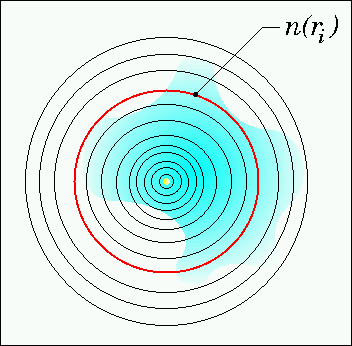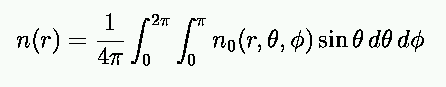Manual - Upload: Density Distribution. General Remarks.If n0(r,theta,phi) is the arbitrary (optically thin) density distribution, the radial density distribution n(r) required for the simulation of the spectral energy distribution can be derived as follows (simply averaging):The quantity r is the radial distance to the illuminating star.

When providing the set of  (r, n(r)) pairs, consider the following:
1. Decrease the distance between two subsequent radial points towards the center (only in case of a constant density this is not required).
2. The step size between to subsequent radial points has to be chosen smaller than the typical size of structures in your density distribution in the particular distance from the star. This is in order not to skip local density enhancements etc. (for the simulation of the spectral energy distribution a linear increase/decrease of the density between two subsequent radial points is assumed!).
Hint: A radial grid with a logarithmic equidistant distribution of grid points is a good choice in case of "smooth" density distribution. In case of a clumpy structure, however, the grid has to be adapted to the size of the clumps in the regions where they are present. The same applies if circumstellar disks with gaps (due to planet-disk interaction) are considered.

See here , if you plan to upload a density distribution resulting from n-particle simulations.